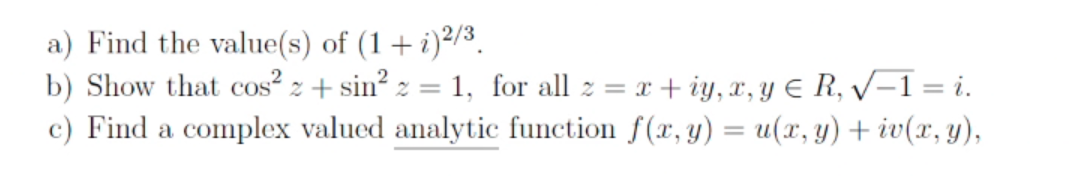### Create an Account

Home / Questions / Find the value(s) of (1 + )2/3. b) Show that cos? z + sin? 2 = 1, for all z = x +iy, , Y E...

# Find the value(s) of (1 + )2/3. b) Show that cos? z + sin? 2 = 1, for all z = x +iy, , Y ER, V-1 = i. c) Find a complex valued analytic function f(x, y) = u(x, y) + iv(x,y),

Find the value(s) of (1 + )2/3. b) Show that cos? z + sin? 2 = 1, for all z = x +iy, , Y ER, V-1 = i. c) Find a complex valued analytic function f(x, y) = u(x, y) + iv(x,y),Jun 03 2021 View more View LessSubscribe To Get Solution